### Home > MC2 > Chapter 5 > Lesson 5.2.2 > Problem5-55

5-55.
1. Simplify the expressions below. Homework Help ✎

1. 52 · (–3) – 4 · 6 + 7

2. –3 · (6 + 4 · 2)

3. 9 + 8 ÷ (–4) –12

4. 23 – 3 · 4 + 6(–1 + 2)

5. 4 + (3 + 4)2

6.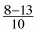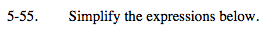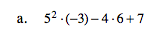Use the order of operations.

Remember, the order begins with parentheses, then exponents, multiplication and division, and lastly, addition and subtraction.

−92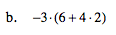Refer to part (a).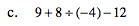Refer to part (a).

−5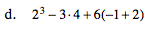Refer to part (a).

2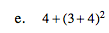Refer to part (a).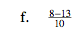Refer to part (a).